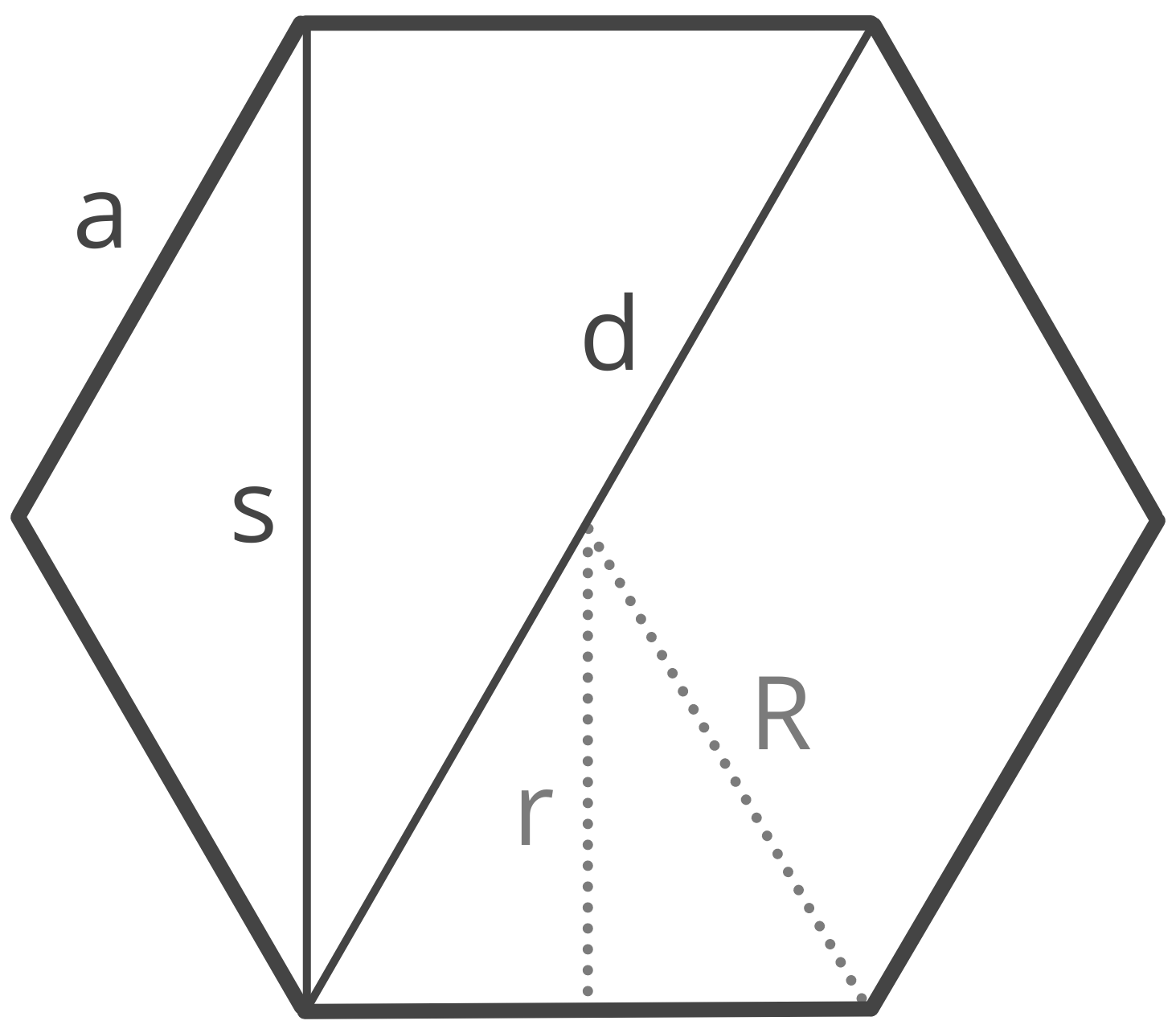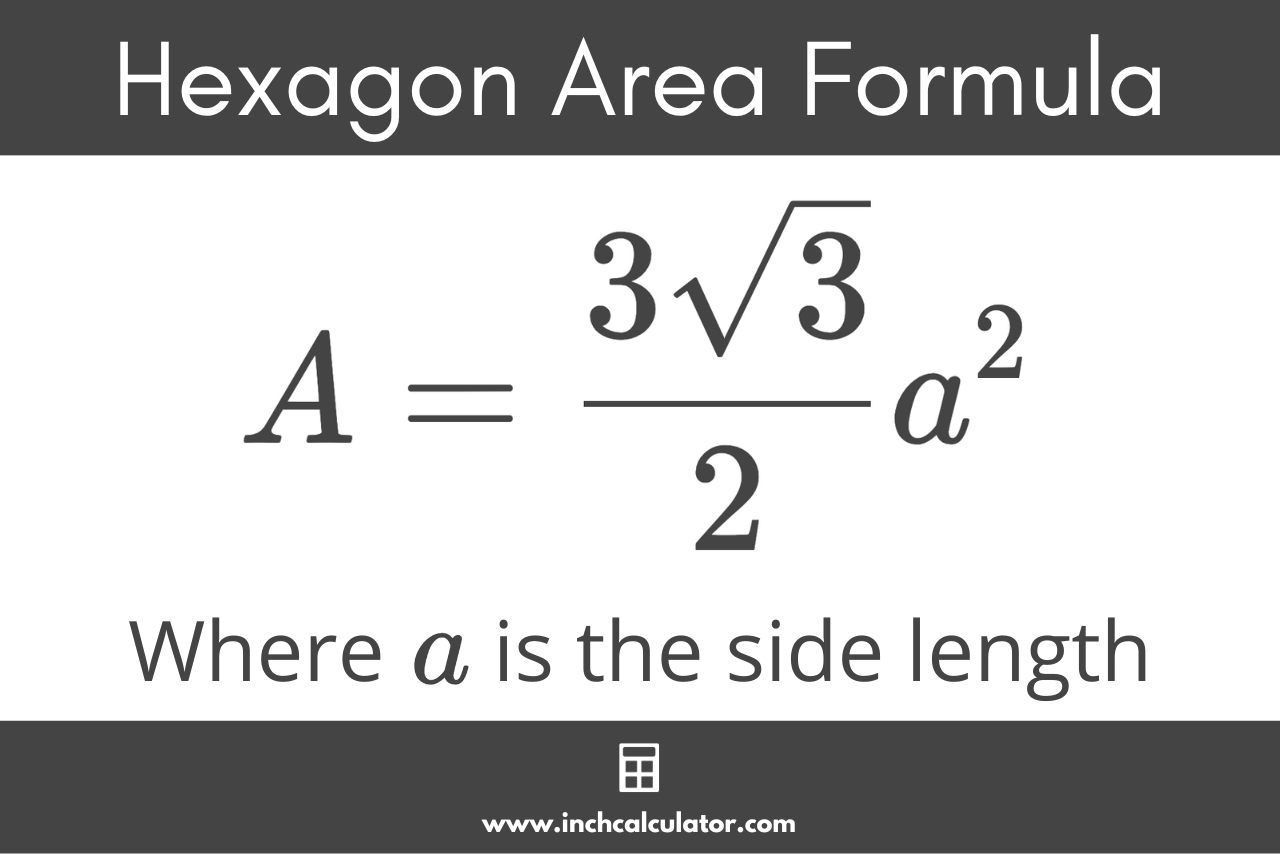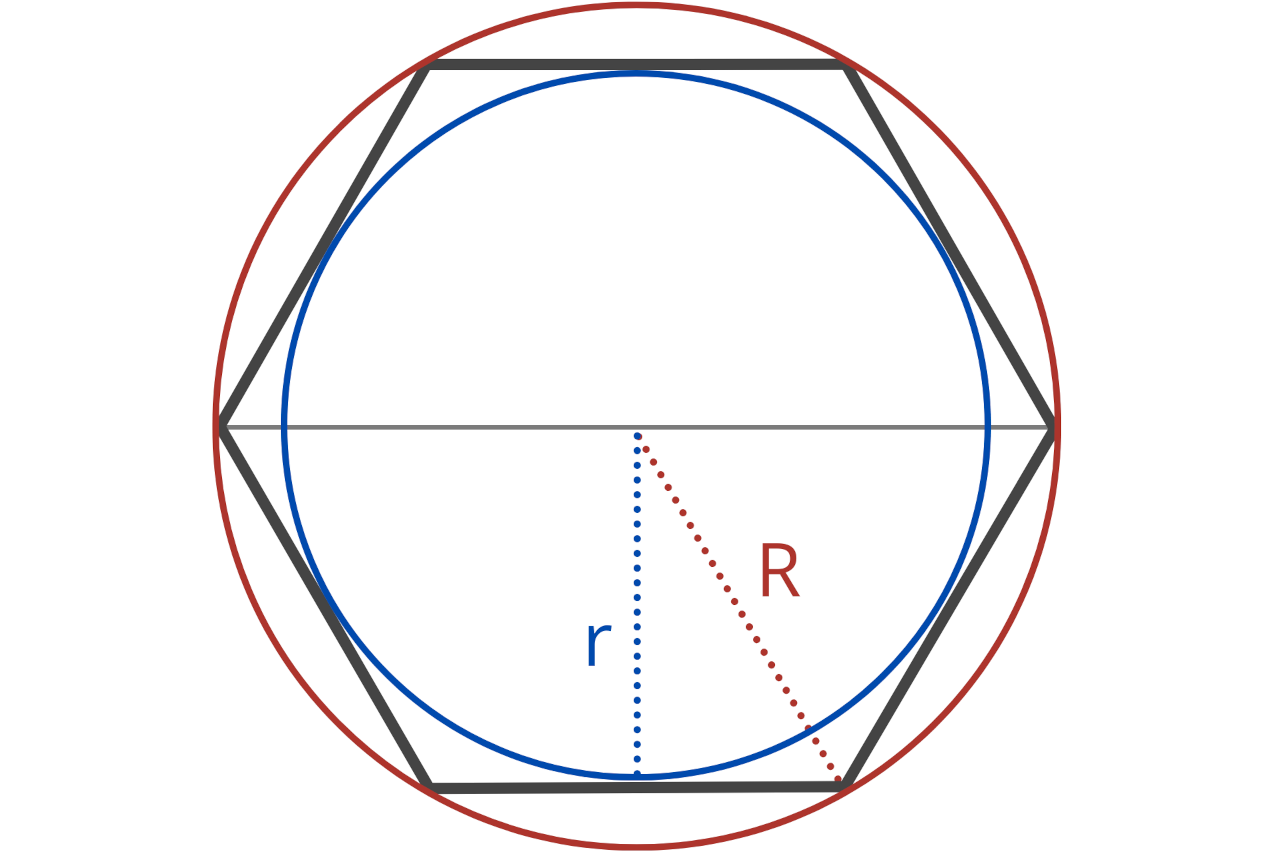# Hexagon Calculator

Calculate any part of a hexagon using the hexagon calculator below.## Solution:

 Side (a): 4 Area (A): 41.569 Perimeter (P): 24 Long Diagonal (d): 8 Short Diagonal (s): 6.928 Circumradius (R): 4 Inradius (r): 3.464
Learn how we calculated this below

## How to Calculate the Properties of a Hexagon

A hexagon is a two-dimensional geometric shape with six straight sides. The properties of a hexagon include the length of the sides a, the area A, the perimeter P, the long diagonal d, the short diagonal s, the radius (circumradius) R, and apothem (inradius) r.

A hexagon is a polygon with six sides and six angles. When all its sides and angles are equal, it’s referred to as a ‘regular’ hexagon. In essence, a regular hexagon is symmetric, with all its internal angles measuring 120 degrees, and it can be divided into six equilateral triangles of equal size.### How to Calculate the Area of a Hexagon

To find the area of a regular hexagon, you can use the hexagon area formula:

A = \frac{3 \sqrt{3}}{2}a^{2}

The area A of a hexagon is equal to 3 times the square root of 3, divided by 2, times the length of the sides a squared.Since a hexagon is composed of six equilateral triangles, this formula is actually derived from the formula for the area of an equilateral triangle. The formula for the area of an equilateral triangle with side length a is:

A_{t} = \frac{\sqrt{3}}{4}a^{2}

The area A of an equilateral triangle is equal to the square root of 3 divided by 4, times the length of the sides a squared.

Since a regular hexagon can be split into six of these triangles, the area of a hexagon is equal to the area of the equilateral triangle times six.

A_{h} = \frac{6 \sqrt{3}}{2}a^{4}

This can be reduced to the following hexagon area formula:

A_{h} = \frac{3 \sqrt{3}}{2}a^{2}

### How to Calculate the Perimeter of a Hexagon

Since a regular hexagon has six equal sides, to find the perimeter, you can multiply the length of the sides by 6.

P = 6a

The perimeter P of a hexagon is equal to 6 times the length of the sides a.

### How to Calculate the Diagonals of a Hexagon

In a regular hexagon:

• A short diagonal connects two non-adjacent vertices that have one vertex between them.
• A long diagonal connects two non-adjacent vertices with two vertices between them.

You can calculate the length of the diagonals using a few formulas.

#### Short Diagonal

The formula to calculate the short diagonal of a regular hexagon is:

s = \sqrt{3} \times a

The short diagonal s is equal to the square root of 3 times the side length a.

#### Long Diagonal

The formula to calculate the long diagonal of a regular hexagon is:

d = 2a

The long diagonal d is equal to twice the side length a.

Just like a triangle or octagon, a hexagon has a circumradius and inradius.

The circumradius of a hexagon is the radius of the circumscribed circle that passes through all the vertices of the hexagon. The circumradius is often referred to as just the radius.

The inradius, or the apothem, of a hexagon is the perpendicular distance from the center of the hexagon to one of its sides. In a regular hexagon, this is also the height of one of the equilateral triangles when split.You can calculate the circumradius and apothem using a few formulas.